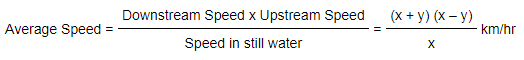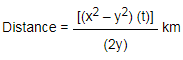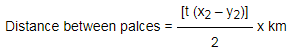# Boats and Streams

#### Video Lesson on Boats and Streams

../aamedia/movie-Set-1/12_boats_and_streams.mp4

Aptitude questions on boats and streams are common in most of the aptitude tests like GAT, NAT, IBA, etc. The advantage with questions on boats and streams is that there are only two basic concepts behind them, and you can solve any questions with these concepts. This article explains how to solve boats and streams problems easily and quickly. The types of questions that can be expected from quantitative aptitude section of boats and streams include following:

### Points to Remember

1. Stream: The moving water of river is called as stream.
2. Still Water: The water which is not moving is known as still water.
3. Upstream: In water, the direction against the stream is known as upstream.
4. Downstream: In water, the direction along the stream is known as downstream.
5. In this chapter the problems are based on the effect of water current on the movement of boats. There are two important phenomena which form the basis for this chapter - upstream (against the water current) & downstream (along with the water current).
If there's no mention of stream speed in the question, assume it to be the speed of boat in still water.
6. Problems similar to boats & streams may also occur which include
a) Cyclist & Wind (where cyclist is analogous to boat while wind is analogous to stream)
b) Swimmer & Stream (where swimmer is analogous to boat)### Important Formulae:

1. If the speed of boat in still water is 'x' km/hr and the speed of stream is 'y' km/hr the Downstream speed (Sd) = (x + y) km/hr
2. IfWhile going downstream, the boat moves in the direction of flow of the river. So, water stream increases the speed of the boat. Hence, speeds are added while going downstream.
Upstream Speed (Su) = (x – y) km/hr
3. If while going upstream, boat moves in the direction opposite to that of flow of river. So, water stream decreases the speed of boat due to opposite flow. Hence, speeds are subtracted while going upstream.
In still water, Speed of boat (x) = ( 1/2 ) x [Downstream speed(Sd) + Upstream speed(Su)]
If we see the above formula closely, we see that adding upstream and downstream speeds gives us 2x, which is twice the speed of the boat, i.e. [x + y + x – y] = 2x.
If you just remember this concept, you won't need to remember and recall the above formula while solving the problems.
4. If you are given speed of the boat upstream and downstream and are required to find out the speed of the current, the following concept is very useful:
Downstream Speed – Upstream Speed = 2 (Speed of the current)
x + y – (x – y) = 2y.
Halving this would give you the speed of the current.
i.e. Speed of stream (y) = (1/2) x [Downstream speed(Sd) – Upstream speed(Su)] 2
5. If a boat moves at 'x' km/hr speed and covers the same distance up and down in a stream of speed 'y' km/hr, then average speed of boat is calculated by,6. If a boat takes time 't' hours more going upstream than to move downstream for the same distance, then the distance is given by,7. If a boat moves to a certain distance downstream in 't1' hours & returns the same distance upstream in time 't2' hours, then8. If a boat takes 't' hours to move a certain place & come back again, then### Boats and Streams Question Types

The problems from this chapter can be divided into four types. Once you know how to deal with these 4 types of problems, the chapter should be an easy one for you.

• Type 1 : Speed of boat in still water and speed of stream are given separately. Find the time taken by boat to go upstream & downstream
• Type 2: Speeds upstream & downstream are given. Find
a) speed of stream
b) speed of boat in still water
• Type 3: Speed of boat in still water & speed of stream are given. Find average speed of boat that covers certain distance & returns through the same path.
• Type 4: Upstream & downstream speeds with respective time are separately given. Find the distance covered by the boat between two points.

### About us

EntryTest.com is a free service for students seeking successful career.CAT - College of Admission Tests. All rights reserved. College of Admission Tests Online Test Preparation The CAT Online